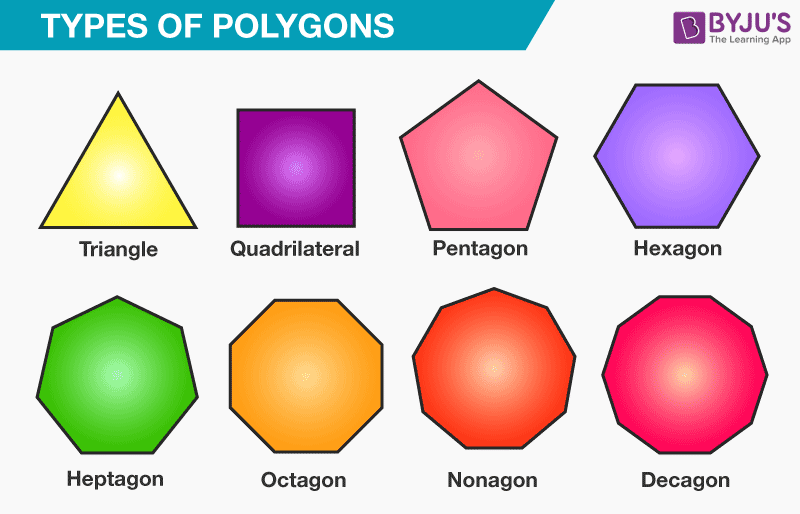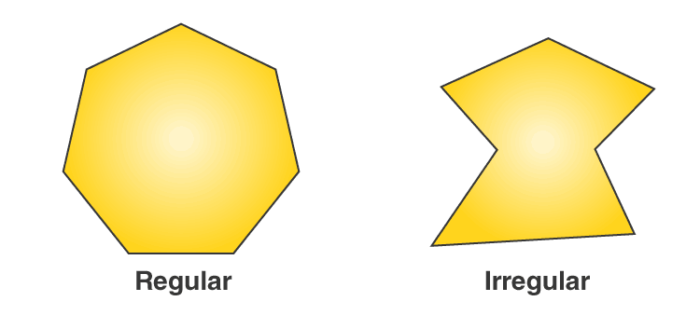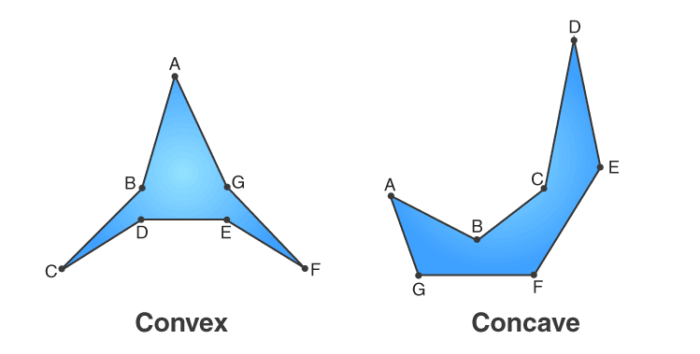Checkout JEE MAINS 2022 Question Paper Analysis : Checkout JEE MAINS 2022 Question Paper Analysis :

# Heptagon

A heptagon is a polygon that has seven sides. It is a closed figure having 7 vertices. A heptagon is also sometimes called Septagon.

In Geometry, the shape that is bounded by at least three straight lines or at least three interior angles is called polygon. The most common examples of polygons are

• Triangle ( 3 sided polygon)
• Quadrilateral ( 4 sided polygon)
• Pentagon ( 5 sided polygon)
• Hexagon ( 6 sided polygon)
• Heptagon ( 7 sided polygon)
• Octagon ( 8 sided polygon) and so on.In this article, let’s discuss the seven-sided polygon called “Heptagon” with proper definition, shape, sides, properties along with its formula in detail.

## Heptagon DefinitionA heptagon is a polygon with 7 sides and 7 angles. Sometimes the heptagon is also known as “septagon”. All the sides of a heptagon meet with each other end to end to form a shape. Therefore,

The number of heptagon sides = 7

## Heptagon shape

Depending on the angles and diagonals, there are different types of heptagon, such as

• Regular and Irregular Heptagon
• Convex and Concave Heptagon

### Regular and Irregular Heptagon

If a heptagon is regular, then all the angles and sides are equal, and the hexagon sides meet each other at an angle of 5π/7 radians or [128(4/7)degrees]. If the heptagon does not have equal side and angle measure, then it is known as irregular heptagon.### Convex and Concave Heptagon

If all the diagonals lie inside the heptagon, it is known as convex heptagon. If some of the diagonals lie outside of the heptagon and one or more interior angles are greater than 180 degrees, then the heptagon is known as concave heptagon.## Heptagon Properties

Some properties of heptagons are as follows:

• In heptagon, the sum of the interior angles is equal to 900 degrees
• The sum of exterior angles of a heptagon is 360 degrees
• For regular heptagon, the measure of the interior angle is about 128.57 degrees
• The measure of the central angle of a regular heptagon is approximately 51.43 degrees
• The number of diagonals in a heptagon is 14
• Regular heptagons are also known as convex heptagons
• The number of triangles formed in a heptagon is 5

## Area of a Heptagon

For a regular heptagon with side length “a”, then the formula to find the area of a heptagon is given as

Area of a heptagon,

$$\begin{array}{l}A = \frac{7}{4}a^{2}\cot \frac{\pi }{7}\end{array}$$
Square units

The above equation is approximately equal to

Area of a Heptagon, A = 3.634a2 square units

## Perimeter of Heptagon

Since all the sides “a” of a regular heptagon are of equal measure, then the perimeter or circumference of a heptagon is written as,

The perimeter of a heptagon, P = 7a units

### Heptagon Solved problem

Question:

Find the area and perimeter of a regular heptagon whose side is 5 cm?

Solution:

Given:

The side of a heptagon, a = 5 cm

We know that

The area of a heptagon, A = 3.634a2 square units

Substitute a = 5 cm in formula,

A = 3.634 (5)2

A = 3.634 (25)

A = 90.85 cm2

Therefore, the area of a heptagon is 90.85 cm2

The perimeter of a heptagon, P = 7a units

P = 7(5)

P = 35 cm

Hence, the perimeter of a heptagon is 35 cm.

Stay tuned with BYJU’S – The Learning App to learn all the interesting Maths concepts and also explore videos to learn with ease.

 Related Links Triangle Circle Octagon Square

### What is a Heptagon in Math?

In maths (geometry), a heptagon is a polygon with seven sides and seven angles. A heptagon has seven straight sides and seven corners i.e. vertices. It is sometimes referred to as a “septagon”.

### What are Regular and Irregular Heptagons?

Regular Heptagons: In a regular heptagon, all the angles and sides are equal.

Irregular Heptagon: In an irregular heptagon, the measure of sides and angles are not equal.

### What are Convex and Concave Heptagons?

Convex Heptagon: In a convex heptagon all its diagonals lies inside it.

Concave Heptagon: In a concave heptagon, one or more interior angles are greater than 180 degrees and some diagonals lie outside the polygon.

### What is the Angle of a Regular Heptagon?

The angle of a regular heptagon is 5π/7 radians or 128.57 degrees. In a heptagon, the sum of all seven angles is 900 degrees.